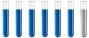## Combustion analysis

Chemistry and homework help forum.

Organic Chemistry, Analytical Chemistry, Biochemistry, Physical Chemistry, Computational Chemistry, Theoretical Chemistry, High School Chemistry, Colledge Chemistry and University Chemistry Forum.

Share your chemistry ideas, discuss chemical problems, ask for help with scientific chemistry questions, inspire others by your chemistry vision!

Please feel free to start a scientific chemistry discussion here!

Discuss chemistry homework problems with experts!

Ask for help with chemical questions and help others with your chemistry knowledge!

Moderators: expert, ChenBeier, Xen

Dhamnekar Winod
Sr. Staff MemberPosts: 172
Joined: Sat Nov 21, 2020 10:14 am
Location: Mumbai[Bombay],Maharashtra State,India

### Combustion analysis

This question presents analytical data from which you are required to determine mass % composition for a compound of up to four elements.

A sample of a compound containing C,H,S and Cl is subjected to combustion analysis to determine the quantities of C and H present. Using a second

sample, the S is analyzed by converting it to BaSO4 The results of the analysis are shown below.

Sample 1 Mass:2.711 ⇒ CO2 Mass:5.070;H2O Mass:2.276

Sample 2 Mass:4.243 ⇒ BaSO4:3.840

Mass of C=Mass of CO2*12/44
5.070*12/44=1.383
% C=1.383/2.711*100= 51.00 %

Mass of H=Mass of H2O*2/18
2.276*2/18=0.253
% H=0.253/2.711*100= 9.33 %

The next element is determined similarly, using the second sample.
Mass of S=Mass of BaSO4*32.06/233.6
3.840 *32.06/233.6=0.5270
% S=0.5270/4.243*100=12.42 %
The final element is obtained by difference.
%Br=100-(51.00 + 9.33 +12.42) = 27.25 %
Any science consists of the following process. 1) See 2)Hear 3)Smell,if needed 4)Taste, if needed 5)Think 6)Understand 7)Inference 8)Take decision [Believe or disbelieve, useful or useless, true or false, cause or effect, any other criteria]
ChenBeier
Distinguished MemberPosts: 798
Joined: Wed Sep 27, 2017 7:25 am
Location: Berlin, Germany

### Re: Combustion analysis

But I thing the goal is to get the formula of that compound